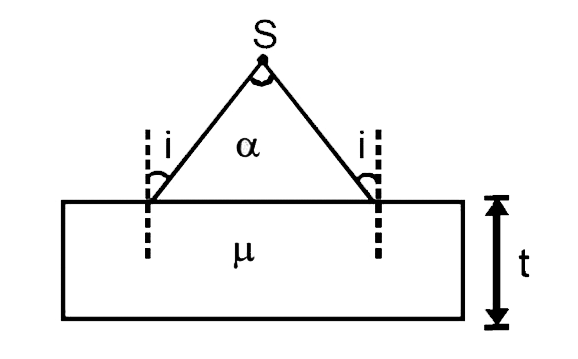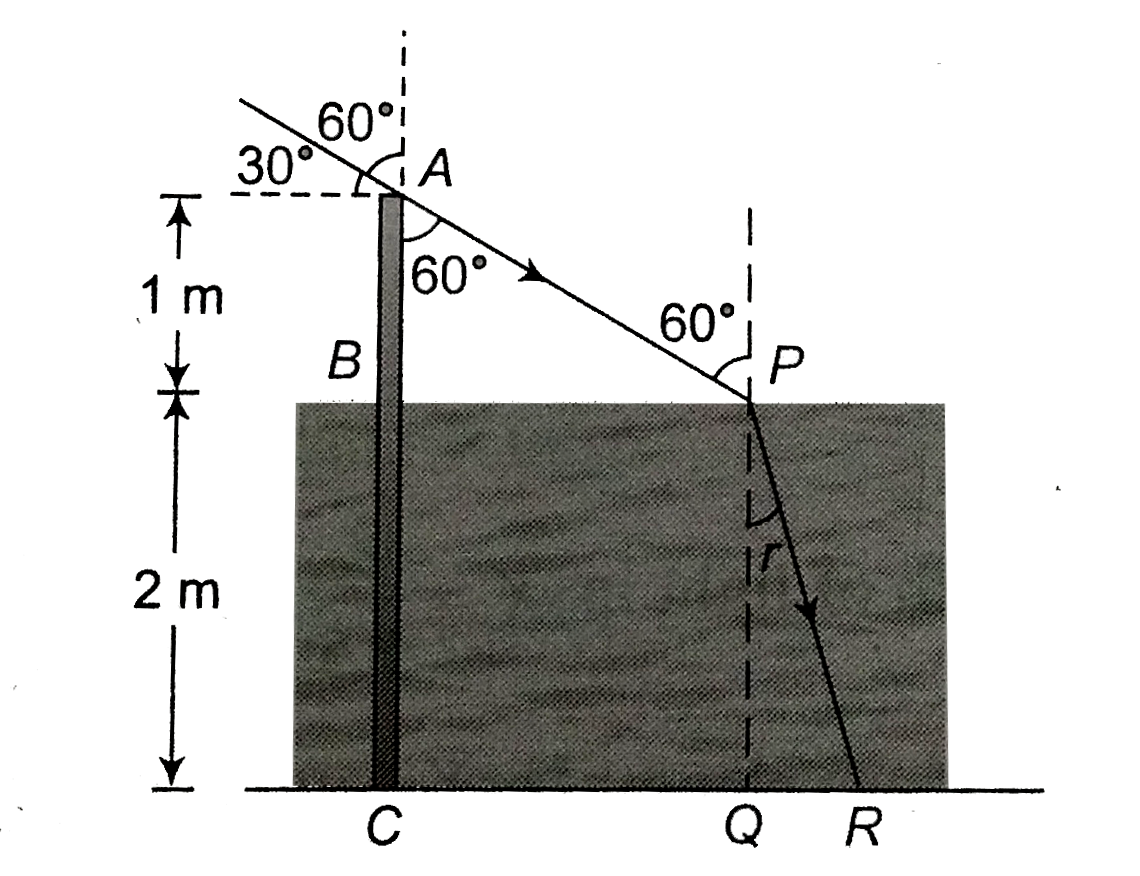# A diverging beam of light from a point source S having devergence angle alpha, falls symmetrically on a glass slab as shown. The angles of incidence

13 views
in Physics
closedA diverging beam of light from a point source S having devergence angle alpha, falls symmetrically on a glass slab as shown. The angles of incidence of the two extreme rays are equal. If the thickness of the glass slab is t and the refractive index n, then the divergence angle of the emergent beam is
A. zero
B. alpha
C. sin^-1(1//n)
D. 2sin^-1(1//n)

by (84.0k points)
selectedi=alpha/2
Divergent angle of emergent beam
=2i=alpha